The definition of the centre of mass, we are now in a position to discuss its physical importance for a system of particles.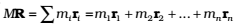…………..(3)

Differentiating the two sides of the equation with respect to time we getOr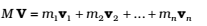…………..(4)

where is the velocity of the first particle, is the velocity of the first particle etc. and V = is the velocity of the centre of mass.

Note that we assumed the masses m1 , m2 , … etc. do not change in time. We have, therefore, treated them as constants in differentiating the equations with respect to time.

Differentiating Eq(4) with respect to time, we obtain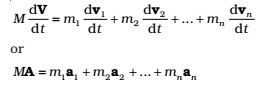…………(5)

where is the acceleration of the first particle, is the acceleration of the first particle etc. and A = is the acceleration of the centre of mass of the system of particles.

Now, from Newton’s second law, the force acting on the first particle is given by F1 = m1a1 .The force acting on the second particle is given by F2 = m2a2 and so on. Eq. (7.9) may be written as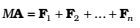The total mass of a system of particles times the acceleration of its centre of mass is the vector sum of all the forces acting on the system of particles.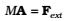………(6)

where Fext represents the sum of all external forces acting on the particles of the system

The centre of mass of a system of particles moves as if all the mass of the system was concentrated at the centre of mass and all the external forces were applied at that point.

Notice, to determine the motion of the centre of mass no knowledge of internal forces of the system of particles is required; for this purpose, we need to know only the external forces.

Click on a star to rate it!

Average rating / 5. Vote count:

No votes so far! Be the first to rate this post.